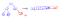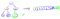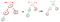# Algorithms on Graphs: Heaps Demystified

Much of the algorithms we’ll be discussing in this series will require us to efficiently pull the lowest values in a set of unsorted numbers without looking at every number in the list.

While sorting or traversing a list may appear to be efficient, it gets rather expensive when we have to do these multiple times, especially when we’re constantly updating or adding new elements to the list.

This is where heaps come into play in helping us get the lowest (or highest) values in a much faster way and in this post, we’ll discuss how.

# What is a heap?

First, let’s talk discuss what a heap exactly is.

Heaps are graphs that have the following properties:

• each node has at most two descending nodes (children nodes)

In the following example, the root node has a value a 3, which is the smallest of all the values in the graph. If we take a look at each subtree, we’ll notice a similar pattern:

Notice that at the root of each subtree, the value is the smallest value of that subtree. This is what we call a min-heap. If the value was instead the largest, we would call this a max-heap.

# Heap Representation

Heaps are commonly represented as array lists. Let’s see how we can translate the visual representation to an array list representation:

There’s a really interesting observation that we make from the array:

From the indices, we can determine the children of each node and vice versa. Here’s an illustration using separate colors to demonstrate which node has which children:

If you haven’t noticed by now, we can use a simple formula to find the index of a child node given the parent’s index.

Assuming index-based 0:

• To find the left child, we use: 2 * parent.index + 1

From the above example:

And what’s great about these equations is that we can use them to find the parents of a given node by simply doing the inverse:

• Find left child’s parent: Math.floor(child.index / 2)

Note: Math.floor() is just a function that drops the decimals of a fraction to give us a whole number

# Insert, Extract, Sift Up, and Sift Down

Great! Now that we know what heaps are and how to find children and parents of each node, we can use these ideas to implement the important functions that are used to maintain a heap’s properties whenever a new node is inserted or extracted.

## Insertion and Sift Up

What’s great about an array list is that we can add new elements to its end at O(1) time. Since we use them to represent heaps, we can use this nice property to add new nodes easily.

This property is great if the place that we add the node maintains the heap properties. But if it doesn’t, we need to a way to move it to the right place.

Suppose that we have the following min-heap and we want to insert a new node:

Parent nodes always add children in the order of left child then right child. This is consistent with our array representation because when we had a new element to the array, we always fill from left to right:Incorrect InsertionCorrect insertion

Now, let’s add another node and suppose that this node is not in the correct position because it is a lower value than the parent.

Let’s take this step by step. When we inserted node 2, we see that it is a lower value than its parent, node 8. In order for it to be in the correct position, we have to make a swap because node 2 needs to be the parent of node 8 in order for the subtree and the overall graph to maintain its heap property.

So now that node 2 is a parent of node 8, we maintained the heap property for that particular subtree but we failed to satisfy the condition for the overall tree because node 2 is less than node 5 but node 5 is the parent of node 2.

So we have to do another swap.

The method that we call on a node to swap with its parent is called sift up.

Here’s the code for siftUp in JavaScript:

Now, I want to address a possible confusion that people (like myself before) might come across when being exposed to heaps for the first time. Let’s look at this situation (suppose we’re trying to make a min-heap):This is not a heap

Here, we have both node 2 and node 3 as values that are less than their parent, node 7. You will never come across this situation because each time a new node is added, the graph must be rearranged to be a heap before another node can be inserted.

That means that before node 3 is inserted, node 2 must first be in the correct place in the heap. So this is what you will see first before node 3 comes into the heap:Put node 2 in the correct position first!

Okay, now that we have got 2 in the correct place, we can now insert node 3 because the graph is a now a heap.

Great! So in summary, we insert one node at a time and sift it around so that it’s in the correct position to maintain the heap property before we can add a new one.

So the algorithm for adding a node to a heap goes as follows:

1. add the node to the end of the array list

Here’s the code for insertNode in JavaScript:

## Analysis of Insert and Sift Up

Since we’re using an array list to represent a heap, adding a new element to the end of it takes O(1) time. However, we need to move it up to its correct place if we wish to maintain the heap.

Remember that the node that’s being sifted up is swapped with its parent. So its index in the array is being divided by 2 (cut in half) after each swap, which means that sifting up takes O(log n) time.

Therefore, inserting and sifting up a node takes O(log n) runtime.

## Extraction and Sift Down

The reason why heaps are so great is that they allow us to extract the minimum (or maximum) value of a set of numbers really quickly.

Let’s think about this scenario. Suppose you had a regular array and you wanted to get and remove the smallest value. You could do two things:

1. Search the array for the smallest value

Both of these operations seem pretty efficient. After all, searching the array just takes O(n) time while sorting takes O(n * log n) (assuming you’re using the most efficient sorting algorithms).

But let’s suppose that instead of doing this just once, you have to do it multiple times. The two operations end up becoming expensive in the long run, especially when values in the array get updated between operations.

This is where heaps shine in getting the smallest (or largest) value in a shorter amount of time. Let’s look at this example of a min-heap:

By definition, the minimum value of a min-heap is the root node. To just get the value, we can simply just call heap and it’ll have O(1) cost. Pretty cool right?

Now let’s suppose we wanted to get the minimum value and remove it from the heap. Instead of pulling from the root directly and go through the trouble of fixing all the remaining nodes to maintain the heap, let’s just swap the root node with the last node:

Since we’re using an array list to represent the heap, we can easily remove the last value of the array list with an O(1) cost.

But we have an issue. The root is no longer the minimum value and because of that, we no longer have a heap.

But don’t panic! We do have a way to correct this. Let’s do something similar to what we did with our siftUp method but in the opposite direction.

Before we can start moving, we need to ask which node is the lowest among node 12’s children? This is important because we need to have the lowest value as the new root in order to maintain the heap. While it’s obvious in the image above, let’s walk through it step by step:

The idea here is that we want to find the smallest values among these three nodes. If 12 > 5, we need to check if 5 is the smallest value by comparing it with 7. Since 5 is not greater than 7, we know that 5 is the smallest value so we swap 12 with 5.

Note: If the root was indeed the smallest value among the three nodes, then it’s already in the correct place and we wouldn’t have to do anything.

And just like siftUp, we apply the same logic going down as we compare 12 with its new children and so on until the overall heap is maintained.

Here’s the code for siftDown:

Here’s the code for extractMin:

## Analysis of Extract and Sift Down:

So extracting the root really boils down to swapping the root with the last node, moving the new root down until we have a new heap and then return the old root.

Since the swapping and extracting takes O(1) time, the bottleneck is really positioning the interim root to its correct place to maintain the heap.

Since siftDown is really just siftUp but going in the opposite direction, the runtime for both extractMin and siftDown is O(log n).

# Summary

The benefit of using heaps is that extracting the minimum (or maximum) value of a list of numbers is relatively faster than traversing the whole list or sorting it. We found that we can do it in O(log n) runtime as opposed to O(n) or O(n * log n) and this difference will save us a lot of time in the long run, especially if we’re asked to repeat this process multiple times in an algorithm.

• Heaps come in two types: min-heaps or max-heaps